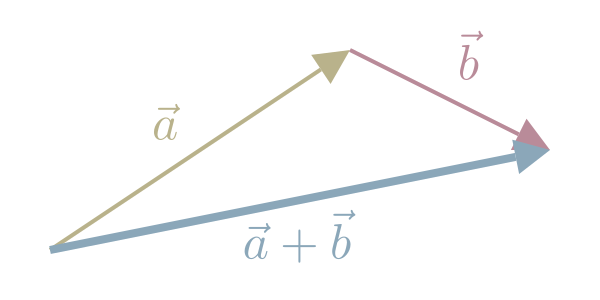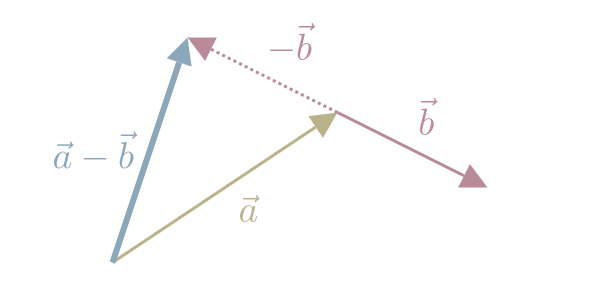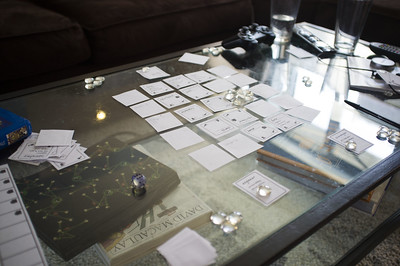• ## Triangularity In Game Design

Schell describes a type of choice in game that is high-risk / high-reward. For example, choosing to shoot the space ship in Space Invaders or taking …

• ## Unified Themes of Games

Schell says that the best games have unified themes in which every element of the game: mechanics, aesthetics, technology and story, each support the theme …$\stackrel{⃗}{a}+\stackrel{⃗}{b}=\left[\begin{array}{c}{a}_{1}\\ {a}_{2}\end{array}\right]+\left[\begin{array}{c}{b}_{1}\\ {b}_{2}\end{array}\right]=\left[\begin{array}{c}{a}_{1}+{b}_{1 \dots }\end{array}$

• ## Vector SubtractionWe subtract one Vector from another by subtracting the corresponding components.

$\stackrel{⃗}{a}-\stackrel{⃗}{b}=\left[\begin{array}{c}{a}_{1}\\ {a}_{2}\end{array}\right]-\left[\begin{array}{c}{b}_{1}\\ {b}_{2}\end{array}\right]=\left[\begin{array}{c}{a}_{1}-{b}_{1 \dots }\end{array}$

• ## Rule of LoopThe Rule of Loop can be summarised in one quote:

"The more times you test and improve your design, the better your game will be …

• ## Expected Value

The sum of weighted outcomes divided by total outcomes

Schell describes Kahneman and Tversky's Prospect Theory as applied to games, in that players will be willing to pay a premium to avoid losses - they …

• ## Newton's Laws Of Motion

In 1686, Newton presented his 3 laws of motion in "Principia Mathematica Philosophiae Naturalis". They are:

• 1st law: an object remains at rest or in …
• ## Flow State

Named by Mihály Csíkszentmihályi in 1975, flow state is the state of being completely immerised in an activity with an "energised focus". Games are examples …

• ## Key Components of Flow For Players

Schell says that the key components that promote Flow State for players are:

• clear goals
• limited distractions
• the "hands and minds" are engaged
• direct and …